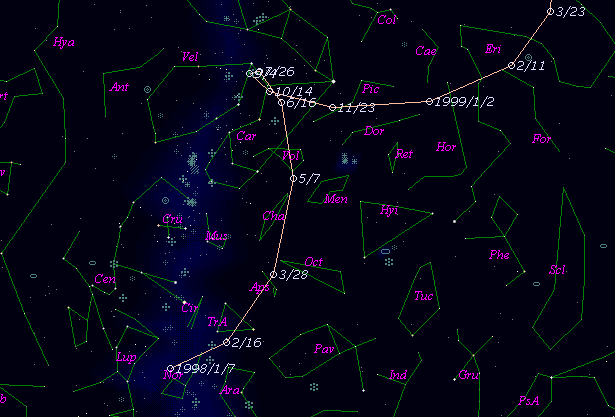\$B%U%'%j%9WB@1(B

C/1999 U1 ( Ferris )\$B%W%m%U%#!<%k(B

 \$BH/8+F|(B 1999\$BG/(B10\$B7n(B18\$BF|(B \$BH/8+8wEY(B 17.3\$BEy(B \$BH/8+ William D. Ferris (Lowell Observatory)\$B\$B50F;MWAG(B

```   The following improved orbital elements by Kenji Muraoka, are
from 125 observations 1999 Oct. 18  to  Dec. 11, perturbations
by 9 Planets, Moon and 5 minor planets were taken into account.
The mean residual is +/- 0.54 arc seconds.

Epoch  =  1998 Aug. 15.0  TT       JDT = 2451040.5
T  =  1998 Sept. 2.87602       +/- 0.27754 (m.e.) TT
Peri. =  291.03258                +/- 0.05868
Node  =   58.25955                +/- 0.02605   (2000.0)
Incl. =  105.72067                +/- 0.03577
q  =    4.1361849              +/- 0.0029835 AU
e  =    1.0030455              +/- 0.0008960
1/a  =   -0.0007363              +/- 0.0002166 1/AU
orig. 1/a  =   +0.000184
fut.  1/a  =   +0.000684
```\$B@1?^(B\$B8wEYJQ2=(B

```        m1 = 6.0 + 5 log\$B&\$(B + 10.0 log r
```\$B50F;MWAG\$OB<2,7r<#;a\$N7W;;\$K\$h\$k\$b\$N\$G\$9!#(B \$B@1?^\$O(B StellaNavigator Ver.2.0 for Windows (\$B%"%9%H%m%"!<%D(B \$BJTCx(B / \$B%"%9%-!<=PHG6I4)(B) \$B\$G:n@.\$7\$?\$b\$N\$G\$9!#(B \$B8wEY%0%i%U\$O(BComet for Windows\$B\$G:n@.\$7\$?\$b\$N\$G\$9!#(B